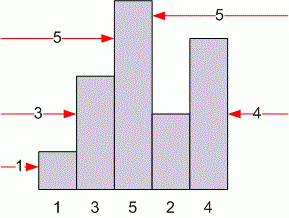Problems

# Skyscrapers

Time limit 1 second
Memory limit 128 MiB

The skyline of the city has n buildings all in a straight line; each building has a distinct height between 1 and n, inclusive. The building at index i is considered visible from the left if there is no building with a smaller index that is taller. Similarly, a building is visible from the right if there is no taller building with a higher index. For example, if the buildings in order are {1, 3, 5, 2, 4}, then three buildings are visible from the left (1, 3, 5), but only two are visible from the right (4 and 5).You will be given the total number of buildings n, l buildings visible from the left, and r buildings visible from the right. Find the number of permutations of the buildings that are consistent with these values.

## Input data

Each line is a separate test case that contains the values of n (1n100), l and r (1l, rn).

## Output data

For each test case print in a separate line the number of permutations of the buildings that are consistent with the given values. The results must be printed modulo 1000000007.

## Examples

Input example #1
4 2 2
5 3 2
8 3 2

Output example #1
6
18
4872